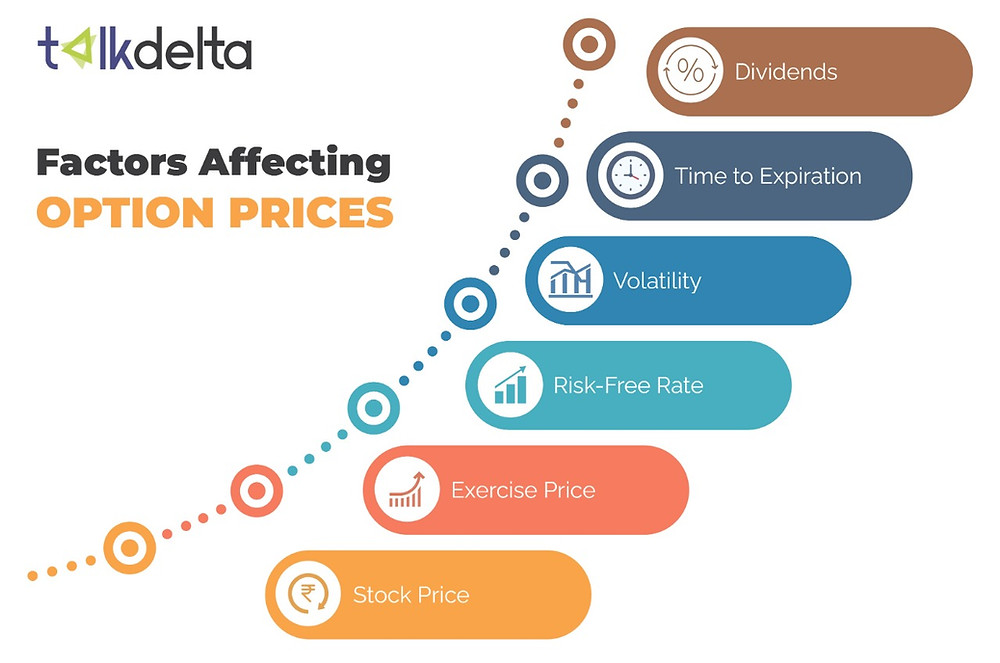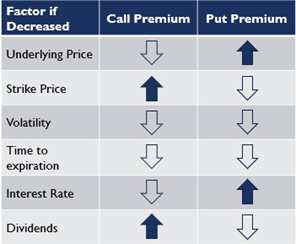Search

# Factors affecting options pricesWho fixes the prices of options? Prices are not fixed by stock exchanges or SEBI or anybody for that matter. In fact, price discovery is a very critical and basic component of markets. Just like a commodity, the prices of options are also influenced by financial and non-financial factors.

Option pricing is the amount per share you have to pay to trade an option. Understanding option pricing is very important for any options trader to make a successful trade. The actual price of an option is determinable when you consider all factors that are responsible for its actual price The Options price is influenced by fluctuating parameters. Let’s discuss the major influencing factors and how do they affect the options prices.

Thus, there are six fundamental parameters that influence the options prices:

• The spot price of the underlying asset

• The strike price of the option

• The volatility of the underlying asset’s price

• Time to expiration

• Interest rates

• Dividends

Refer to the images below for better inference of the below explained factors and their effects on the options prices.Spot Price

The spot price is the price of the underlying asset. The prices of the options are derived on the basis of the value of their underlying asset. The spot price is a major factor that influences the options pricing as the current value or price of the option's underlying instrument has a direct effect on the price of the call or put option.

If the value of the underlying instrument is on the rise then the call option price will increase and the put option price will decrease.

If the price of the underlying instrument decreases then the call option price will decrease and the put option price will increase.

Strike Price

Strike Price is the price at which a derivative contract can be bought or sold when it is exercised. The position of the strike price is relative to the underlying price of the asset and this has an impact on the price of an option because it directly impacts the intrinsic value of an option.

If all the other factors remain constant but the strike price of options increases, the intrinsic value (premium) of the call option will decrease and the intrinsic value of the put option will increase.

On the other hand, with all the other factors remaining constant, a decrease in the strike price of the option will increase the intrinsic value (premium) of the call option and decrease the intrinsic value (premium) of the put option.

Volatility

This factor plays a major role in affecting the options prices. Volatility is the difference recorded in day-to-day stock prices. It is the swing in the underlying asset’s price, either up or down. It affects both call and put options in the same way.

Higher the volatility of the underlying stock, the higher the premium because there is a greater possibility that the option will move in the money (ITM) during the life of the contract.

Higher volatility = Higher premium (for both call and put options)

Lower volatility = Lower premium (for both call and put options)

If the volatility is on the rise then the call option price will increase and the put option price will also increase. If the volatility falls then the call option price and the put option price, will decrease.

Time to Expiration

The effect of the Time to expiration factor on the prices of the options is the same as that of Volatility. The longer the maturity of the option, the greater is the uncertainty and hence higher is the premium.

If all other factors affecting an option’s price remain the same, the time value portion of an option’s premium will decrease with the passage of time. This is also known as time decay. Options are known as ‘wasting assets’, due to this property where the time value gradually falls to zero.

If the Time to Expiration is on the rise then the call option price and the put option price, both will increase. If the Time to Expiration falls then the call option price and the put option price, both will decrease.

Interest Rates

Interest rates are slightly complicated because they affect different options, differently. For example, interest rates have a greater impact on options with individual stocks and indices compared to options on futures.

To put it in a simpler way, high interest rates will result in an increase in the value of a call option and a decrease in the value of a put option.

On the other hand, lower interest rates will result in a decrease in the value of a call option and an increase in the value of a put option.

Dividends

In the event of dividend announcements during the life of an option, the exchanges adjust the option positions. When the stock trades and still its holder gets no dividends, the situation is termed ex-dividend. As per regulations by SEBI, if the value of the dividend is more than 10% of the spot price of the option on the date the dividend is announced, then the strike price of the options is reduced by the dividend amount on ex-dividend dates. For dividends announced lower than 10% of the spot price, there is no adjustment by the exchange.

A dividend announcement decreases the value of the call option as the stock value decreases on the ex-dividend date, and increases the value of the put option. This is vice versa if the dividend factor is not in the picture.

CONCLUSION

Changes in any or all of these variables affect the option's value. These factors facilitate theoretically value an option. This helps the options trader to decide on the options as per your investment goal. Options factors analysis provides flexibility to tailor the risk and reward of every trade as per your individual strategies.

“Losses are necessary, as long as they are associated with a technique to help you learn from them”